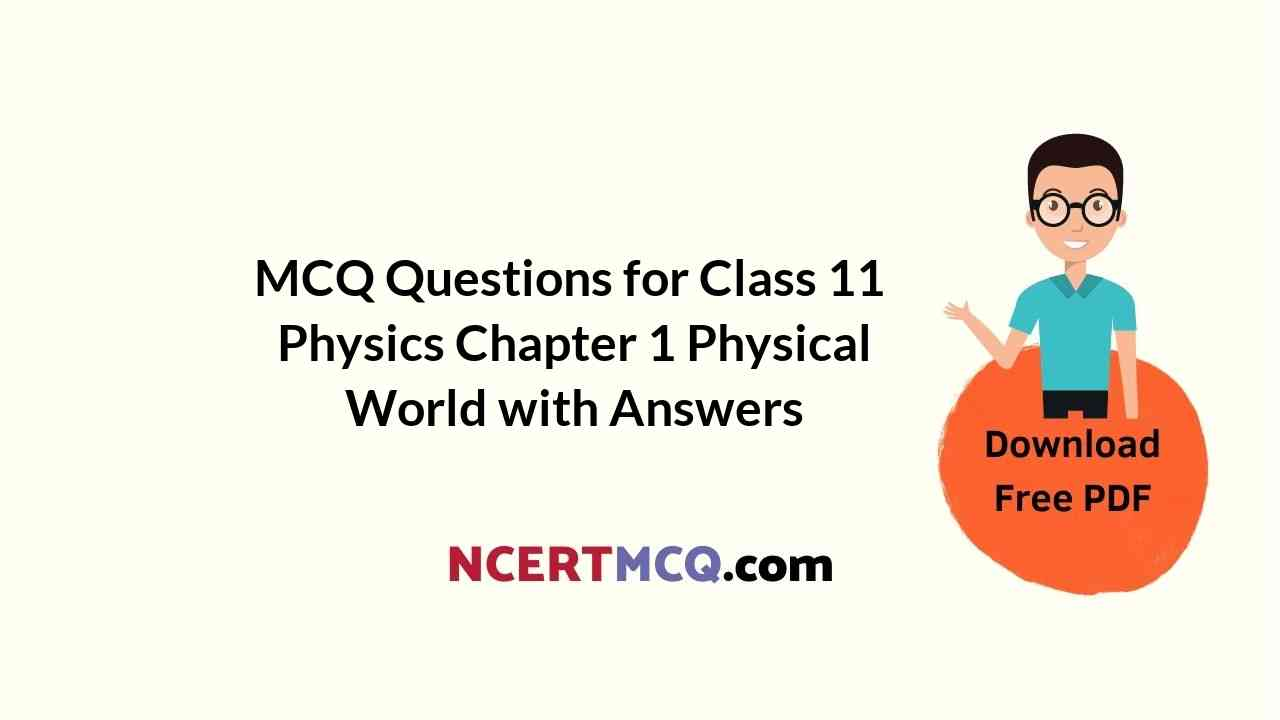Check the below NCERT MCQ Questions for Class 11 Physics Chapter 1 Physical World with Answers Pdf free download. MCQ Questions for Class 11 Physics with Answers were prepared based on the latest exam pattern. We have provided Physical World Class 11 Physics MCQs Questions with Answers to help students understand the concept very well. https://ncertmcq.com/mcq-questions-for-class-11-physics-with-answers/

## Physical World Class 11 MCQs Questions with Answers

MCQ Questions For Class 11 Physics Chapter 1 Question 1.
The word Science originates from the Latin verb Scientia meaning
(a) to know
(b) to see
(c) to experience
(d) to observe

Class 11 Physics Chapter 1 MCQ Question 2.
Atomic and molecular phenomena are dealt with by
(a) Newtonian Mechanics
(b) fluid Mechanics
(c) applied Mechanics
(d) Quantum Mechanics

Physical World Class 11 MCQ Questions Question 3.
Wave picture of light failed to explain.
(a) the photoelectric effect
(b) polarization of light
(c) diffraction of light
(d) interference of light

Class 11 Physics Chapter 1 MCQ With Answers Question 4.
Heliocentric theory proposed by Nicolas Copernicus was
(a) replaced by circular orbits to fit the data better
(b) replaced by elliptical orbits to fit the data better
(c) replaced by elliptical orbits to fit the taste of new rulers of Italy
(d) replaced by parabolic orbits to fit the data better

Answer: (b) replaced by elliptical orbits to fit the data better

Physical World Class 11 MCQ Question 5.
Just as a new experiment may suggest an alternative theoretical model, a theoretical advance may suggest what to look for in some for in some experiments. Which of the following experiments can be considered to support this claim?
(a) Davisson and Germer Experiment
(b) experimental discovery of positron
(c) scattering of alpha particle or the gold foil experiment
(d) Michelson Morley experiment

Answer: (b) experimental discovery of positron

Physics Class 11 Chapter 1 MCQ Questions Question 6.
The scientific method is
(a) a prescribed method for investigating phenomena, acquiring new knowledge…
(b) A procedure for proposing new hypothesis
(c) a body of techniques for investigating phenomena, acquiring new knowledge…
(d) A method for proposing new theories.

Answer: (c) a body of techniques for investigating phenomena, acquiring new knowledge…

MCQ On Physical World Class 11 Question 7.
A scientific theory
(a) cannot be changed but can be reformulated
(b) is fixed once and for all because it is logical
(c) is changed to suit new fashion among scientists
(d) can be revised if required to fit new phenomenon or data

Answer: (d) can be revised if required to fit new phenomenon or data

Ch 1 Physics Class 11 MCQ Question 8.
Which of the following is a possible first step in applying the scientific method
(a) Conducting tests
(b) Formulating a hypothesis
(c) Formulation of a question
(d) Building a theory

Answer: (c) Formulation of a question

Class 11 Physics Ch 1 MCQ Question 9.
Which of the following is a possible final step in applying the scientific method
(a) Formulating a hypothesis
(b) Building a theory
(c) Analysis of test results
(d) Formulation of a question

Answer: (c) Analysis of test results

MCQs Of Physics Class 11 Chapter 1 With Answers Question 10.
Physics is a
(a) Applied Science
(b) Mathematical Science
(c) Engineering Science
(d) Natural Science

Physics Class 11 Chapter 1 MCQ Question 11.
Newtonian mechanics could not explain
(a) fall of bodies on earth
(b) Some of the most basic features of atomic phenomena.
(c) movement of planets
(d) flight of rockets